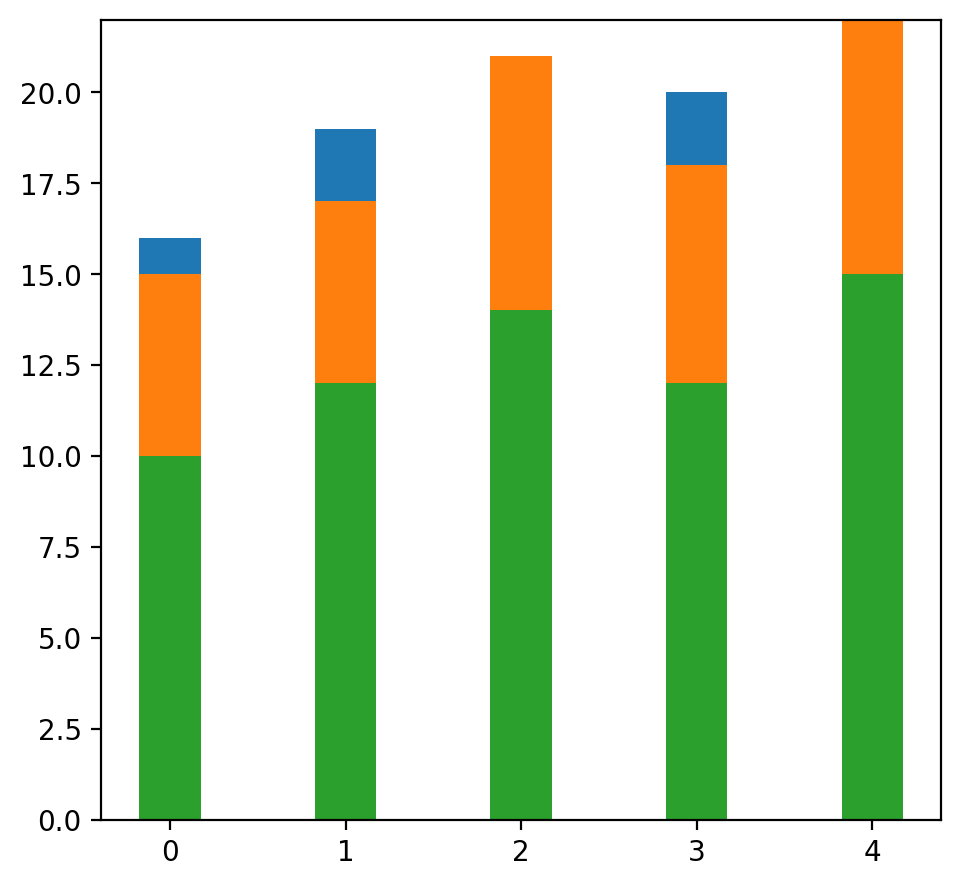## Matplotlib Tutorial: Histograms and Bar Plots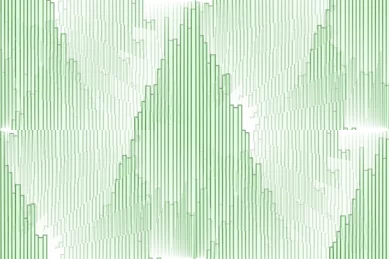It's hard to imagine that you open a newspaper or magazin without seeing some bar charts or histograms telling you about the number of smokers in certain age groups, the number of births per year and so on. It's a great way to depict facts without having to use too many words, but on the downside they can be used to manipulate or lie with statistics as well. They provide us with quantitative information on a wide range of topics. Bar charts and column charts clearly show us the ranking of our top politicians. They also inform about consequences of certain behavior: smoking or not smoking. Advantages and disadvantages of various activities. Income distributions and so on. On the one hand, they serve as a source of information for us to see our own thinking and acting in statistical comparison with others, on the other hand they also - by perceiving them - change our thinking and acting in many cases.

However, we are primarily interested in how to create charts and histograms in this chapter. A splendid way to create such charts consists in using Python in combination with Matplotlib.

What is a histogram? A formal definition can be: It's a graphical representation of a frequency distribution of some numerical data. Rectangles with equal width have heights with the associated frequencies.

If we construct a histogram, we start with distributing the range of possible x values into usually equal sized and adjacent intervals or bins.

We start now with a practical Python program. We create a histogram with random numbers:

import matplotlib.pyplot as plt
import numpy as np
gaussian_numbers = np.random.normal(size=10000)
gaussian_numbers

Output:
array([-0.80404388, -2.77483865, -2.26510843, ..., -0.6985082 ,
1.21066701,  0.4700107 ])
plt.hist(gaussian_numbers)
plt.title("Gaussian Histogram")
plt.xlabel("Value")
plt.ylabel("Frequency")
plt.show()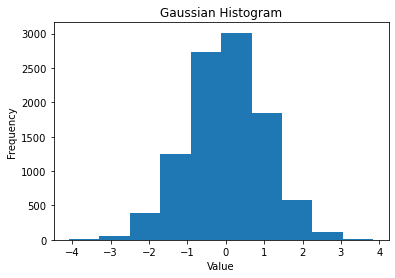We have seen that the function hist (actually matplotlib.pyplot.hist) computes the histogram values and plots the graph. It also returns a tuple of three objects (n, bins, patches):
n, bins, patches = plt.hist(gaussian_numbers)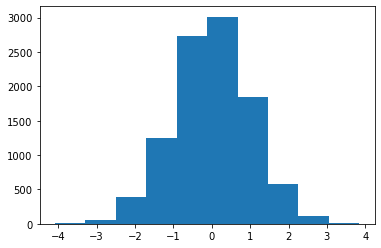n[i] contains the number of values of gaussian numbers that lie within the interval with the boundaries bins [i] and bins [i + 1]:

print("n: ", n, sum(n))

n:  [   5.   61.  385. 1254. 2728. 3012. 1839.  585.  115.   16.] 10000.0


So n is an array of frequencies. The last return value of hist is a list of patches, which corresponds to the rectangles with their properties:

print("patches: ", patches)
for i in range(10):
print(patches[i])

patches:  <BarContainer object of 10 artists>
Rectangle(xy=(-4.08653, 0), width=0.79308, height=5, angle=0)
Rectangle(xy=(-3.29345, 0), width=0.79308, height=61, angle=0)
Rectangle(xy=(-2.50037, 0), width=0.79308, height=385, angle=0)
Rectangle(xy=(-1.70729, 0), width=0.79308, height=1254, angle=0)
Rectangle(xy=(-0.914206, 0), width=0.79308, height=2728, angle=0)
Rectangle(xy=(-0.121126, 0), width=0.79308, height=3012, angle=0)
Rectangle(xy=(0.671954, 0), width=0.79308, height=1839, angle=0)
Rectangle(xy=(1.46503, 0), width=0.79308, height=585, angle=0)
Rectangle(xy=(2.25811, 0), width=0.79308, height=115, angle=0)
Rectangle(xy=(3.05119, 0), width=0.79308, height=16, angle=0)


Let's take a closer look at the return values. To create the histogram array gaussian_numbers are divided into equal intervals, i.e. the "bins". The interval limits calculated by hist are obtained in the second component of the return tuple. In our example, they are denoted by the variable bins:

n, bins, patches = plt.hist(gaussian_numbers)
print("n: ", n, sum(n))
print("bins: ", bins)
for i in range(len(bins)-1):
print(bins[i+1] -bins[i])
print("patches: ", patches)
print(patches)
print(patches)

n:  [   5.   61.  385. 1254. 2728. 3012. 1839.  585.  115.   16.] 10000.0
bins:  [-4.08652622 -3.29344612 -2.50036601 -1.7072859  -0.9142058  -0.12112569
0.67195442  1.46503452  2.25811463  3.05119474  3.84427484]
0.7930801067329991
0.7930801067329987
0.7930801067329987
0.7930801067329991
0.7930801067329991
0.7930801067329982
0.7930801067329991
0.7930801067329991
0.7930801067329991
0.7930801067329982
patches:  <BarContainer object of 10 artists>
Rectangle(xy=(-3.29345, 0), width=0.79308, height=61, angle=0)
Rectangle(xy=(-2.50037, 0), width=0.79308, height=385, angle=0)Let's increase the number of bins. 10 bins is not a lot, if you imagine, that we have 10,000 random values. To do so, we set the keyword parameter bins to 100:

plt.hist(gaussian_numbers, bins=100)
plt.show()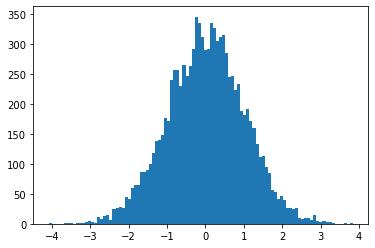Indem wir den Parameter orientation auf vertical setzen, können wir das Histogramm auch seitwärts ausgeben:

plt.hist(gaussian_numbers,
bins=100,
orientation="horizontal")
plt.show()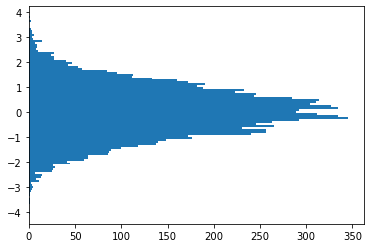Another important keyword parameter of hist is density, which replaces the deprecated normed parameter. If set to true, the first component - that is, the frequencies - of the return tuple is normalized to form a probability density, i. the area (or the integral) under the histogram makes the sum 1

n, bins, patches = plt.hist(gaussian_numbers,
bins=100,
density=True)
plt.show()
print("Area below the integral: ", np.sum(n * np.diff(bins)))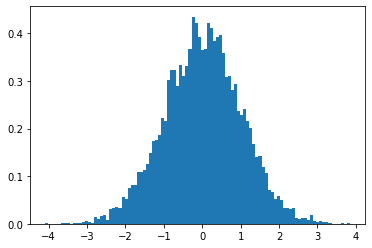Area below the integral:  1.0


If both the parameters 'density' and 'stacked' are set to 'True', the sum of the histograms is normalized to 1. With the parameters edgecolor and color we can define the line color and the color of the surfaces:

plt.hist(gaussian_numbers,
bins=100,
density=True,
stacked=True,
edgecolor="#6A9662",
color="#DDFFDD")
plt.show()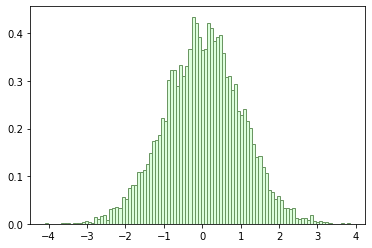Okay, you want to see the data depicted as a plot of cumulative values? We can plot it as a cumulative distribution function by setting the parameter 'cumulative'.

plt.hist(gaussian_numbers,
bins=100,
stacked=True,
cumulative=True)

plt.show()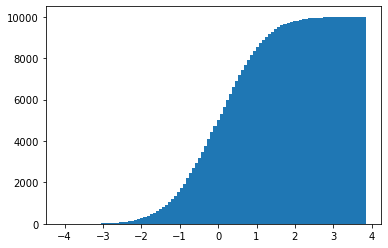### Bar Plots

Now we come to one of the most commonly used chart types, well known even among non-scientists. A bar chart is composed of rectangles that are perpendicular to the x-axis and that rise up like columns. The width of the rectangles has no mathematical meaning.

bars = plt.bar([1, 2, 3, 4], [1, 4, 9, 16])
bars.set_color('green')
plt.show()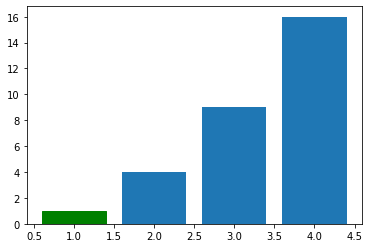f=plt.figure()
ax.bar([1,2,3,4], [1,4,9,16])
children = ax.get_children()
children.set_color('g')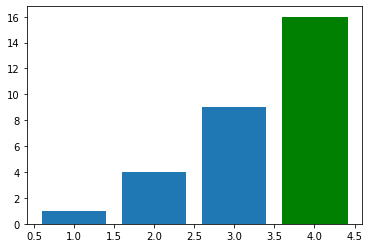import matplotlib.pyplot as plt
import numpy as np
years = [str(year) for year in range(2010, 2021)]
visitors = (1241, 50927, 162242, 222093, 665004,
2071987, 2460407, 3799215, 5399000, 5475016, 6003672)
index = np.arange(len(years))
bar_width = 0.9
plt.bar(index, visitors, bar_width,  color="green")
plt.xticks(index, years) # labels get centered
plt.show()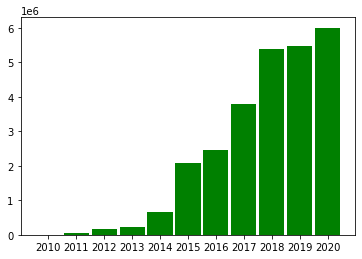### Barplots with Customized Ticks

from matplotlib.ticker import FuncFormatter
import matplotlib.pyplot as plt
import numpy as np

def millions(x, pos):
'The two args are the value and tick position'
#return '$%1.1fM' % (x * 1e-6) return f'${x * 1e-6:1.1f}M'
formatter = FuncFormatter(millions)

years = ('US', 'EU', 'China', 'Japan',
'Germany', 'UK', 'France', 'India')
GDP = (20494050, 18750052, 13407398, 4971929,
4000386, 2828644, 2775252, 2716746)

fig, ax = plt.subplots()
ax.yaxis.set_major_formatter(formatter)
ax.bar(x=np.arange(len(GDP)), # The x coordinates of the bars.
height=GDP, # the height(s) of the vars
color="green",
align="center",
tick_label=years)
ax.set_ylabel('GDP in ') ax.set_title('Largest Economies by nominal GDP in 2018') plt.show()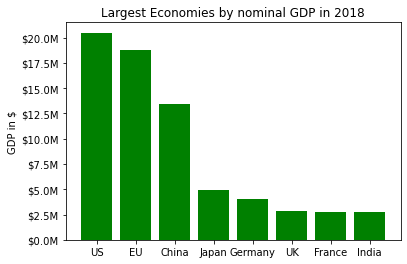The file 'data/GDP.txt' contains a listing of countries, GDP and the population numbers of 2018 in the following format: 1 United States 20,494,050 326,766,748 — European Union 18,750,052 511,522,671 2 China 13,407,398 1,415,045,928 3 Japan 4,971,929 127,185,332 4 Germany 4,000,386 82,293,457 5 United Kingdom 2,828,644 66,573,504 6 France 2,775,252 65,233,271 7 India 2,716,746 1,354,051,854 8 Italy 2,072,201 59,290,969 9 Brazil 1,868,184 210,867,954 10 Canada 1,711,387 36,953,765 11 Russia 1,630,659 143,964,709 12 South Korea 1,619,424 51,164,435 13 Spain 1,425,865 46,397,452 14 Australia 1,418,275 24,772,247 15 Mexico 1,223,359 130,759,074 16 Indonesia 1,022,454 266,794,980 17 Netherlands 912,899 17,084,459 18 Saudi Arabia 782,483 33,554,343 19 Turkey 766,428 81,916,871 20 Switzerland 703,750 8,544,034 Create a bar plot with the per capita nominal GDP. import matplotlib.pyplot as plt import numpy as np land_GDP_per_capita = [] with open('data/GDP.txt') as fh: for line in fh: index, *land, gdp, population = line.split() land = " ".join(land) gdp = int(gdp.replace(',', '')) population = int(population.replace(',', '')) per_capita = int(round(gdp * 1000000 / population, 0)) land_GDP_per_capita.append((land, per_capita)) land_GDP_per_capita.sort(key=lambda x: x, reverse=True) countries, GDP_per_capita = zip(*land_GDP_per_capita) fig = plt.figure(figsize=(6,5), dpi=200) left, bottom, width, height = 0.1, 0.3, 0.8, 0.6 ax = fig.add_axes([left, bottom, width, height]) ax.bar(x=np.arange(len(GDP_per_capita)), # The x coordinates of the bars. height=GDP_per_capita, # the height(s) of the vars color="green", align="center", tick_label=countries) ax.set_ylabel('in thousands of')
#ax.set_xticks(rotation='vertical')
ax.set_title('Largest Economies by nominal GDP in 2018')
plt.xticks(rotation=90)
plt.show()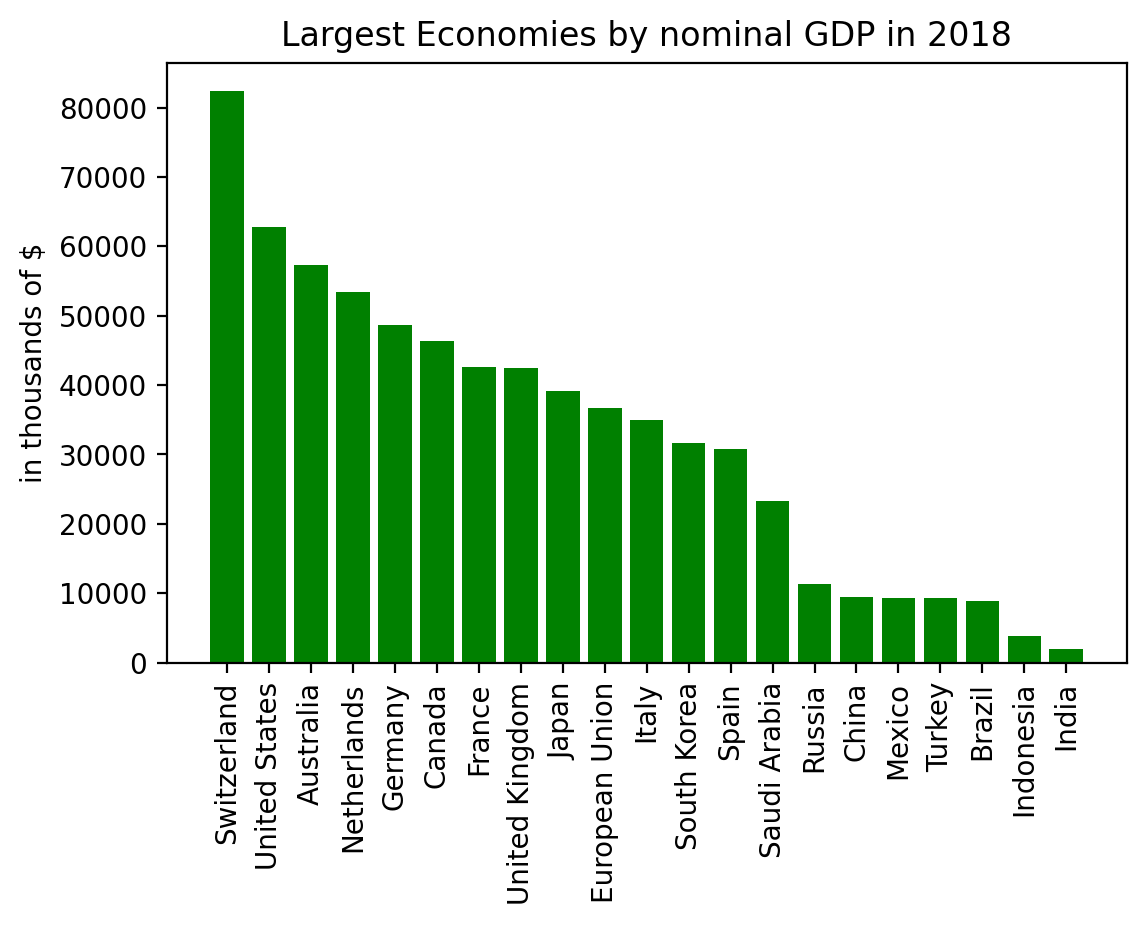### Vertical Bar Charts (Line Charts)

import matplotlib.pyplot as plt
import numpy as np
import matplotlib.pyplot as plt
# restore default parameters:
plt.rcdefaults()
fig, ax = plt.subplots()
personen = ('Michael', 'Dorothea', 'Robert', 'Bea', 'Uli')
y_pos = np.arange(len(personen))
cups = (15, 22, 24, 39, 12)
ax.barh(y_pos, cups, align='center',
color='green', ecolor='black')
ax.set_yticks(y_pos)
ax.set_yticklabels(personen)
ax.invert_yaxis()
ax.set_xlabel('Cups')
ax.set_title('Coffee Consumption')
plt.show()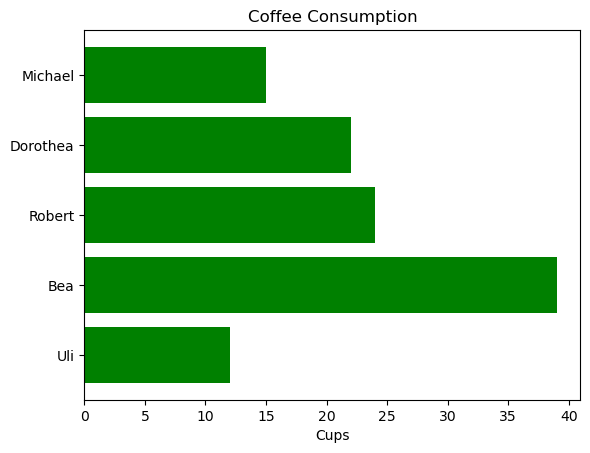### Grouped Bar Charts

So far we used in our bar plots for each categorical group one bar. I.e., for each country in the previous example we had one bar for the "per capita GDP" of one year. We could think of a graph representing these values for different years. This can be accomplished with grouped bar charts. A grouped bar chart contains two or more bars for each categorical group. These bars are color-coded to represent a particular grouping. For example, a business owner, running a production line with two main products might make a grouped bar chart with different colored bars to represent each product. The horizontal axis would show the months of the year and the vertical axis would show the revenue.

import matplotlib.pyplot as plt
import numpy as np

last_week_cups = (20, 35, 30, 35, 27)
this_week_cups = (25, 32, 34, 20, 25)
names = ['Mary', 'Paul', 'Billy', 'Franka', 'Stephan']

fig = plt.figure(figsize=(6,5), dpi=200)
left, bottom, width, height = 0.1, 0.3, 0.8, 0.6
ax = fig.add_axes([left, bottom, width, height])

width = 0.35
ticks = np.arange(len(names))
ax.bar(ticks, last_week_cups, width, label='Last week')
ax.bar(ticks + width, this_week_cups, width, align="center",
label='This week')

ax.set_ylabel('Cups of Coffee')
ax.set_title('Coffee Consummation')
ax.set_xticks(ticks + width/2)
ax.set_xticklabels(names)

ax.legend(loc='best')
plt.show()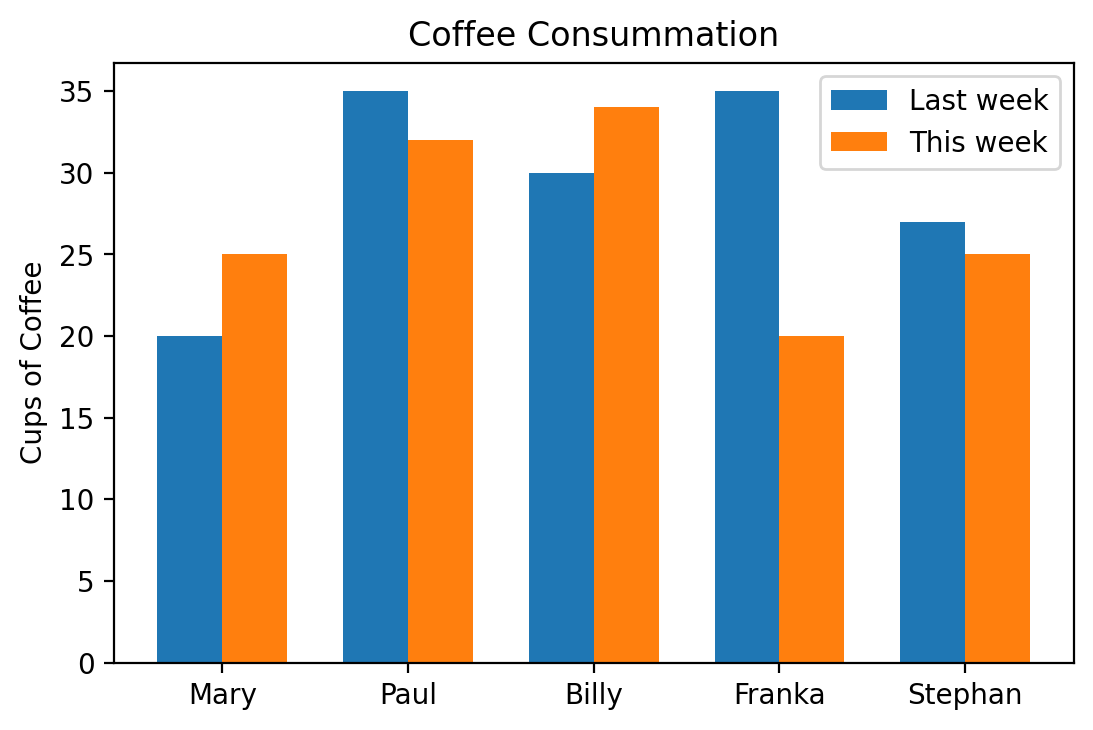### Exercise

The file data/german_election_results.txt contains four election result of Germany.

Create a bar chart graph with data.

import matplotlib.pyplot as plt
import numpy as np

parties = ('CDU/CSU', 'SPD', 'FDP', 'Grüne', 'Die Linke', 'AfD')

election_results_per_year = {}
with open('data/german_election_results.txt') as fh:
for line in fh:
year, *results = line.rsplit()
election_results_per_year[year] = [float(x) for x in results]

election_results_per_party = list(zip(*election_results_per_year.values()))

fig = plt.figure(figsize=(6,5), dpi=200)
left, bottom, width, height = 0.1, 0.1, 0.8, 0.8
ax = fig.add_axes([left, bottom, width, height])

years = list(election_results_per_year.keys())
width = 0.9 / len(parties)
ticks = np.arange(len(years))

for index, party in enumerate(parties):
ax.bar(ticks+index*width, election_results_per_party[index], width, label=party)

ax.set_title('German Elections')
ax.set_xticks(ticks + 0.45)
ax.set_xticklabels(years)

ax.legend(loc='best')
plt.show()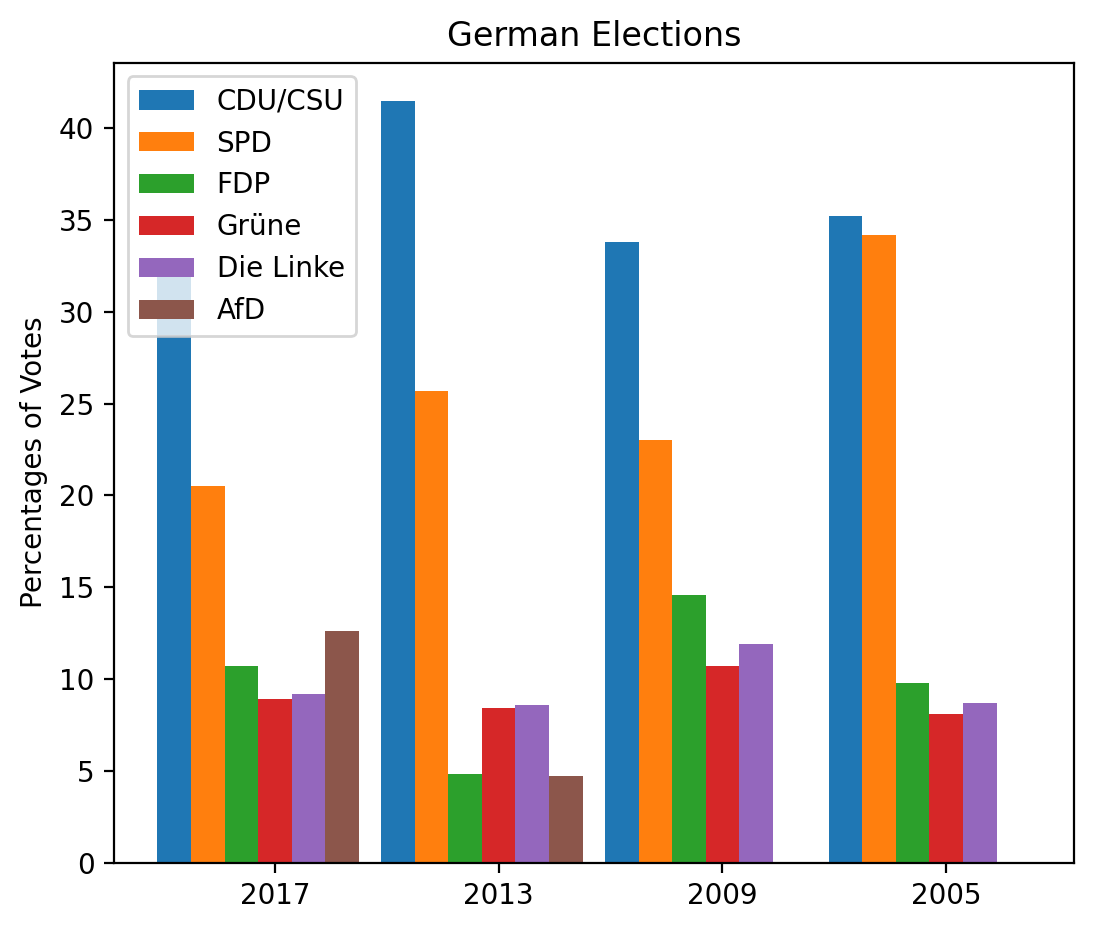We change the previous code by adding the following dictionary:

colors = {'CDU/CSU': "black", 'SPD': "r", 'FDP': "y",
'Grüne': "green", 'Die Linke': "purple", 'AfD': "blue"}


We also change the creation of the bar code by assigning a color value to the parameter ‚color‘:

for index, party in enumerate(parties):
ax.bar(ticks+index*width,
election_results_per_party[index],
width,
label=party,
color=colors[parties[index]])


Now the complete program with the customized colors:

import matplotlib.pyplot as plt
import numpy as np

parties = ('CDU/CSU', 'SPD', 'FDP', 'Grüne', 'Die Linke', 'AfD')
colors = {'CDU/CSU': "black", 'SPD': "r", 'FDP': "y",
'Grüne': "green", 'Die Linke': "purple", 'AfD': "blue"}

election_results_per_year = {}
with open('data/german_election_results.txt') as fh:
for line in fh:
year, *results = line.rsplit()
election_results_per_year[year] = [float(x) for x in results]

election_results_per_party = list(zip(*election_results_per_year.values()))

fig = plt.figure(figsize=(6,5), dpi=200)
left, bottom, width, height = 0.1, 0.1, 0.8, 0.8
ax = fig.add_axes([left, bottom, width, height])

years = list(election_results_per_year.keys())
width = 0.9 / len(parties)
ticks = np.arange(len(years))

for index, party in enumerate(parties):
ax.bar(ticks+index*width,
election_results_per_party[index],
width,
label=party,
color=colors[parties[index]])

ax.set_title('German Elections')
ax.set_xticks(ticks + 0.45)
ax.set_xticklabels(years)

ax.legend(loc='best')
plt.show()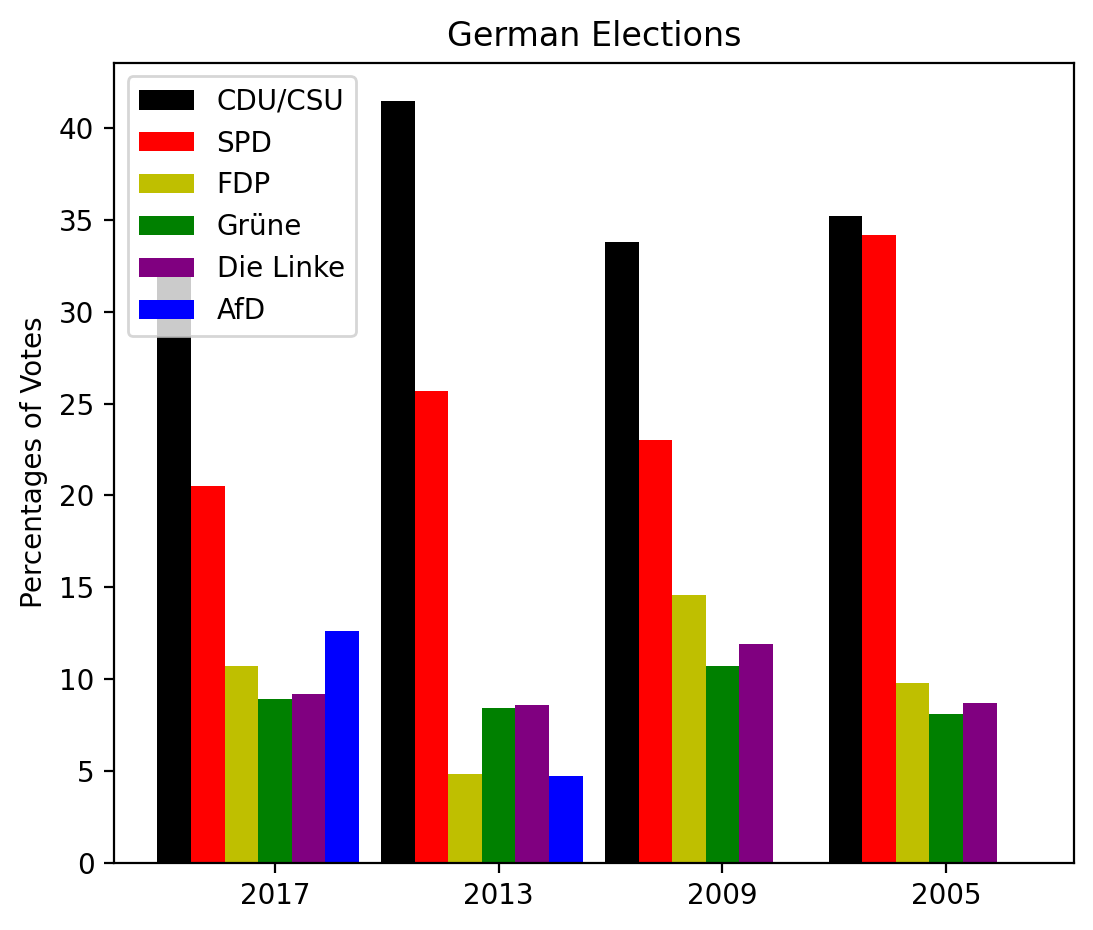#### Stacked Bar Chars

As an alternative to grouped bar charts stacked bar charts can be used.

The stacked bar chart stacks bars that represent different groups on top of each other. The height of the resulting bar shows the combined result or summation of the individual groups.

Stacked bar charts are great to depict the total and at the same time providing a view of how the single parts are related to the sum.

Stacked bar charts are not suited for datasets where some groups have negative values. In such cases, grouped bar charts are the better choice.

import matplotlib.pyplot as plt
import numpy as np

coffee = np.array([5, 5, 7, 6, 7])
tea = np.array([1, 2, 0, 2, 0])
water = np.array([10, 12, 14, 12, 15])
names = ['Mary', 'Paul', 'Billy', 'Franka', 'Stephan']

fig = plt.figure(figsize=(6,5), dpi=200)
left, bottom, width, height = 0.2, 0.1, 0.7, 0.8
ax = fig.add_axes([left, bottom, width, height])

width = 0.35
ticks = np.arange(len(names))
ax.bar(ticks, tea, width, label='Coffee', bottom=water+coffee)
ax.bar(ticks, coffee, width, align="center", label='Tea',
bottom=water)
ax.bar(ticks, water, width, align="center", label='Water')

Output:
<BarContainer object of 5 artists>